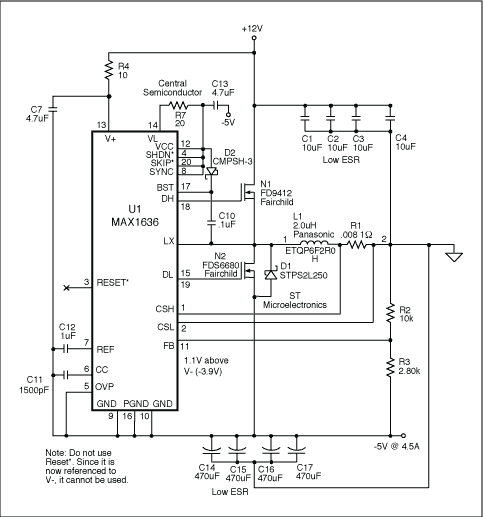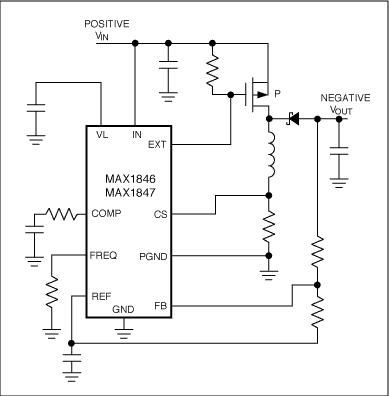# Buck Regulator Forms High-Power Inverting -5V Supply

### Abstract

Configuring a step-down, switching-converter IC as an inverter yields an efficient, high-powered, -5V supply capable of outputting currents up to 4.5A (12V input) or 3.2A (5V input). The circuit can be modified to deliver other voltage levels.

### Introduction

Configuring a step-down switching-converter IC as an inverter (Figure 1) yields an efficient, high-powered -5V supply, capable of outputting currents up to 4.5A (12V input) or 3.2A (5V input). With changes in component values, the circuit can deliver other voltages.Conventional inverting power supplies do the switching using a p-channel MOSFET (Figure 2). That configuration works well at lower currents, but has limited use above around 2A, depending on the input and output voltage levels and the p-channel MOSFET being used. Comparing a standard buck circuit (Figure 3) with Figure 1, you see that the converter's "output" in Figure 1 connects to ground, and (what used to be) ground becomes the -5V output.Because the on-resistance of an n-channel MOSFET is generally lower than that of comparable p-channel devices, a power supply with n-channel MOSFETs usually provides more current at higher efficiency. To turn on, however, an n-channel MOSFET requires a gate voltage approximately 4V higher than its source (usually the supply voltage). Several high-power step-down converters include small charge pumps (comparable to D2 and C10 in Figure 1) for generating the elevated gate voltage.

The Figure 1 circuit achieves high output current and high efficiency by reconfiguring a high-power buck converter (U1) as an inverter to take advantage of its all-n-channel-MOSFET design. Efficiency is 90% with a 12.35V input, -5.02V output, and 4.7A load, or 84% with a 4.56V input, -5.02V output, and 3.3A load. You can easily accommodate -5.2V applications by changing the values of R2 and R3, or both. (Operation at -5.2V incurs a small penalty on maximum output current.)

Input and output ripple voltages are directly related to the input and output capacitors' equivalent series resistance (ESR), so those capacitors should be chosen carefully. Circuit layout is also extremely important (as for all dc-dc converters). You may want to consider an evaluation kit from Maxim (MAX1636EVKit) that includes a small circuit board with optimized layout and all components necessary for operating the MAX1636. Because its layout is similar to the one required in Figure 1, the EVKit can serve as a rough layout guide for this design idea.

This design idea appeared in the April 1, 2004 issue of EDN magazine.